## General Time Dependent Perturbations

Assume that we solve the unperturbed energy eigenvalue problem exactly:. Now we add a perturbation that depends on time,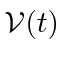. Our problem is now inherently time dependent so we go back to the time dependent Schrödinger equation.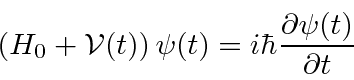We will expandin terms of the eigenfunctions:with. The time dependent Schrödinger equations isNow dotinto this equation to get the time dependence of one coefficient.Assume that at, we are in an initial stateand hence all the other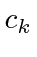are equal to zero:.Now we want to calculate transition rates. To first order, all theare small compared to, so the sum can be neglected.This is the equation to use to compute transition probabilities for a general time dependent perturbation. We will also use it as a basis to compute transition rates for the specific problem of harmonic potentials. Again we are assumingis small enough that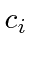has not changed much. This is not a limitation. We can deal with the decrease of the population of the initial state later.

Note that, if there is a large energy difference between the initial and final states, a slowly varying perturbation can average to zero. We will find that the perturbation will need frequency components compatible withto cause transitions.

If the first order term is zero or higher accuracy is required, the second order term can be computed. In second order, a transition can be made to an intermediate state, then a transition to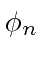. We just put the first order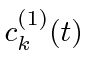into the sum.* Example: Transitions of a 1D harmonic oscillator in a transient E field.*

Jim Branson 2013-04-22